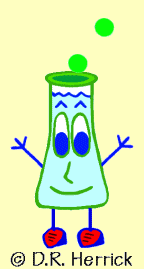Dr. Herrick's CH221 Pressure Quiz 6Quantum Theory Copyright D. Herrick20 Questions from past exams. Practice for speed.  Aim for 2 minutes per problem.

 Constants: Avogadro's number:   NA = 6.02 × 1023 / mole speed of light:  c = 3.00 × 108 m/s Planck's constant:  h = 6.63 × 10-34 J-s electron volt:  1 eV  = 1.60 × 10-19 J calorie:  1 cal = 4.184 J Angstrom:  1 Å = 1 × 10-10 m = 100 pm

 1 Which wavelength of light has the highest frequency?
 A) 1000Å B) 800nm C) 0.001cm D) 0.01mm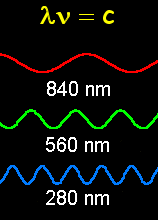2 Calculate the frequency (s-1) of green light of wavelength 5000Å
 A) 6.85 × 1015 B) 2.34 × 1015 C) 0.60 × 1015 D) 1.28 × 1015 E) 0.49 × 1015
 3 The energy (J) of one green photon of wavelength 5000Å is
 A) 2.14 × 10-19 B) 3.36 × 10-19 C) 9.98 × 10-18 D) 1.67 × 10-19 E) 3.98 × 10-19
 4 The energy (kJ) of one mole of green photons of wavelength 5000Å is
 A) 240 B) 132 C) 216 D) 306 E) 347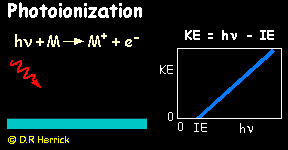5 The energy in J/photon of blue light at a wavelength of 424.0 nm is
 A) 6.28 × 10-11 B) 4.69 × 10-19 C) 7.07 × 10-20 D) 6.42 × 10-17 E) 2.81 × 10-38
 6 According to Planck the energy of a photon is
 A) proportional to the square of the radiation frequency B) proportional to the inverse of the wavelength C) independent of the color of the radiation D) proportional to the inverse square of the frequency E) proportional to the wavelength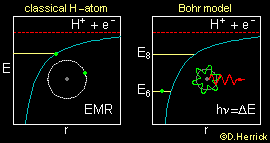7 The initial step in reactions that deplete atmospheric ozone is photodissociation of a chlorofluorocarbon molecule at a wavelength of 200 nm,CF2Cl2 + light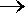CF2Cl + Cl The energy of this radiation in kJ per mole of photons is
 A) 178 B) 39 C) 854 D) 599 E) 361
 8 Which is NOT consistent with the Bohr theory?
 A) The classical picture of an electron moving in a circular orbit around the nucleus is replaced by a standing wave for the electron. B) The energy of an electron is  quantized. C) An electron may move to a lower orbit by emitting radiation of frequency proportional to the energy difference between the two orbits. D) The radius of an orbit increases at higher energy levels. E) The energy of an orbit is independent of the nuclear charge.
 9 The Bohr radius of hydrogen in the ground state is 0.529Å.  The radius of a hydrogen atom with n = 7 is
 A) 92.6Å B) 3.70Å C) 25.9Å D) 0.529Å E) 52.9Å10 The Bohr radius of hydrogen in the ground state is 52.9pm.  The Bohr radius of a helium ion He+ in the ground state is
 A) 212pm B) 26.5pm C) 13.2pm E) 52.9pm E) 106pm
 11 The radius of a Bohr orbit in terms of the quantum number n and the nuclear charge Z is proportional to
 A)  n 2 / Z B)  n2 / Z 2 C)  n / Z 2 D)  n / Z
 12 The energy to ionize an electron from a Bohr orbit is proportional to
 A) Z/n2 B) Z2 / n C) Z 2 / n 2 D) Z / n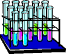13 According to deBroglie the quantum wavelength of a particle of mass m moving with velocity v is
 A) proportional to (mv) B) proportional to the square of (mv) C) independent of (mv) D) proportional to the inverse of (mv) E) proportional to the inverse square of (mv)
 14 Which is an allowed set of quantum numbers  (n, l, m)  for the hydrogen atom?
 A) (4, -2, 1) B) (24, 12, -14) C) (8, 8, 8) D) (213, 210, 198) E) (11, -5, -5)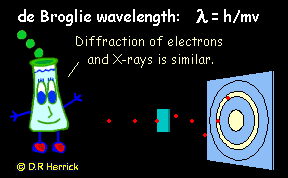15 In which orbital does the electron in a hydrogen atom have the largest  ionization energy?
 A) 3p D) 9d B) 8d E) 7p C) 2s F) 15f
 16 In which orbital does the electron in a hydrogen atom have the largest angular momentum?
 A) 10s D) 23p B) 8f E) 34d C) 5d F) 9p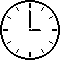17 How many spatial orbitals are there in the 5f  subshell?
 A) 7 D) 5 B) 25 E) 15 C) 75 F) 35
 18 The allowed values of the magnetic quantum number for spatial orbitals with n = 3 in the H atom are
 A) 0,1,2 D) 3,2,1,0,-1,-2,-3 B) 0,1,2,4 E) -1,0,1 C) -2,-1,0,1,2 F) 0,1,2,3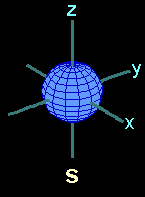19 What is the total number of spatial orbitals with principal quantum number 4 in the C5+ ion?
 A) 9 B) 25 C) 80 D) 49 E) 16
 20 Light emitted from the transition 3d2p in the hydrogen atom has l = 656 nm.  What is the wavelength of light from the transition 500f499d in hydrogen?
 A) 5680 nm F) 5.68 mm B) 123 pm G) 47.1 mm C) 47.1 cm H) 568 cm D) 56.8 nm I) 1.23 mm E) 12.3 m J) 4.71 m